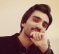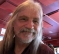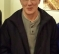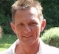## Choosing the DFT size to match linear convolution in overlap save method?Started by 4 years ago11 replieslatest reply 4 years ago790 views

I am studying DFT from S.K.Mitra's book and I am now trying to write MATLAB code for the overlap save method (a.k.a overlap discard).

It works just the way it should according to the book. I get some zeros at the beginning because I am not doing an additional step, which I'll incorporate later. My question is about the end of the output.

I get zeros at the end, depending on the length of the DFT denoted by N in the code. But with direct linear convolution, there are no zeros at the end. Is it supposed to be this way?

Also, with direct convolution the output length is N+M-1, whereas with my code the length is the same as the lengthy sequence x. Again, is it supposed to be this way?

I'm pretty sure my code implements the equations for overlap save correctly.

I'd be grateful for any guidance. Thanks.

Following is my code

>> x = randi([0,3],1,73);
>> h = [2 1 0 1 2];
>> yl = overlap_save(x,h);

function yl = overlap_save(x,h)
M = numel(h);
overlap = M - 1;
N = 2*overlap;
h = [h,zeros(1,N-M)];
H = fft(h);
NT = numel(x);
step = N-overlap;
m = 0;
yl = zeros(1,NT);
while((N + m*step)<=NT)
xm = x((1:N) + m*step);
yc = ifft(fft(xm).*H);
yl((M:N) + m*step) = yc(M:N);
m = m+1;
end
[ - ]Hi szak1592.

I don't think your code is working properly. And I don't have time right now to examine your code in detail. But I do have a few suggestions for you.

 Change your output variable name from 'yl' to 'yL'. (Having any MATLAB variable name end with a lowercase letter 'L' will make people go crazy when they try to read your code. That's because an 'l' letter looks almost identical to the number '1'.)

 Change your input sequence to

x = 1:20;

so that your filter output results will NOT be random each time you run your code. That makes debugging easier.

 If your input sequence is x = 1:20;, the correct yL output sequence will be:

yL =

Columns 1 through 8

2  5  8  12  18  24  30  36

Columns 9 through 16

42  48  54  60  66  72  78  84

Columns 17 through 24

90  96  102  108  72  55  58  40

 I have an "Overlap & Save' MATLAB routine from many years ago. I don't recall if I wrote this routine myself, or if I stole it (fair-and-square) from someone else. Note in my code that the FFT size MUST be larger than the length of the impulse response (your variable 'h') of the filter. In any case, the following MATLAB routine might be of some help to you:

% Filename: FastConv_OverlapAndSave_test.m
%
%    Used to test the "OverlapAndSave.m" function
%    for implementing "fast convolution".
%
%    Richard Lyons, August 2005

clear, clc
%  *** Define input & overlap and save (OLS) parameters ***
%X = sin(2*pi*7.88*(0:127)/128 + pi/3);  % Filter input sequence
%X = 3:21; X = [X,0,fliplr(X)];
%h = [1,1,22,1];

N = 6;  % Fast Conv. FFT size. MUST BE LARGER THAN filter imp response
%X = randi([0,3],1,73); % szak1592's random input
X = 1:20;
h = [2 1 0 1 2]; % szak1592's filter imp. response

Len_input = length(X)
Len_h = length(h)

Y_true = conv(X,h);  % Standard time-domain convolution result

%  *** Implement overlap and save (OLS) algorithm ***
[Y] = FastConv_OverlapAndSavex(X,h,N); % Call the OLS function

Len_FastConvOutput = length(Y)
Len_MatlabConvOutput = length(Y_true)

%  *** Plot and compare OLS with standard time-dom. convolution ***
Time_X = 0:length(X)-1;  % Time-domain index for plotting
Time_Y = 0:length(Y)-1;  % Time-domain index for plotting

figure(1)
subplot(2,1,1)
plot(Time_X,X,'-ko','markersize',2), grid on, zoom on
title('Filter input sequence')
subplot(2,1,2)
plot(Time_Y,Y,'-ro','markersize',5)
hold on
Time_Y_true = 0:length(Y_true)-1;  % Time-domain index for plotting
plot(Time_Y_true,Y_true,'b*','markersize',3)
hold off, grid on, zoom on
title('Results: Red = Fast Conv, Blue = True time-domain Conv')
xlabel('Time')

function [y] = FastConv_OverlapAndSavex(x,h,N)
%  [y] = FastConv_OverlapAndSave(x,h,N)
%  Overlap-and-save method of "fast convolution" filtering
%  of long time-domain sequences.
%
%  Partitions the x input sequence into multiple blocks and
%  uses the FFT to implement high speed time-domain convolution.
%
%  x = filter input time-domain sequence
%  h = impulse response (coefficients) of a FIR filter
%  N = FFT size
%  y = filter output time-domain sequence
%
%    Example:
%        x = 3:21; x = [x,0,fliplr(x)];  % Input time sequence
%        h = [1,0,-1];                   % Filter imp. response (differentiator)
%        N = 8;                          % FFT size
%        [y] = FastConv_OverlapAndSave(x,h,N)
%
%    Richard Lyons, August 2005

P = length(x);  %  Length of time sequence x.
Q = length(h);  %  Length of filter impulse response.
L = N-(Q-1);

h(N) = 0;     % Zero pad FIR filter imp. response to N samples
H = fft(h);   % Filter freq response

x = [zeros(1,Q-1), x, zeros(1,N-1)]; % Append zeros to beginning and end of x.

NumBlocks = floor((P + Q-2)/(L)) + 1; % Number of data blocks
y = [];  % Initialize output vector

for J = 1:NumBlocks
Xblock_J = x((J-1)*L+1:(J-1)*L+N);         % Current block of x(n) data
CircConv_J = ifft(H.*fft(Xblock_J));       % Current circ convolution result
y = [y, CircConv_J(Q:length(CircConv_J))]; % Concatinate output data blocks
end

y = y(1:P+Q-1);
end

[ - ]Thank you, Rick Lyons. This is very helpful. I'll go through it, see where I went wrong and how I can modify my code accordingly.

Thanks.

[ - ]Hey there szak1592,

I have to admit I haven't implemented your code and thus I cannot be 100% sure about the reasons it is not working the way it should.

Nevertheless, it seems to me that the issue is at the value of 'NT'. Correct me if I am wrong but I think that, the way you have coded it, the final iteration of the code will not be executed (because your "index" will exceed the value of NT) and thus the final part of your convolved signal will not be calculated.

The reason is that you set NT equal to the number of elements in 'x' (your original signal) while it should be equal to [x + overlap] (if I have understood the code correctly).

Again, I apologize for not giving a definite answer to your question. I will try to implement and debug your code as soon as I find some time and let you know of my findings.

Best,

Achilles.

EDIT

Holy...!!!

I am really sorry... I thought we were talking about overlap-add... I am extremely sorry for that... :(

Well, I did take a closer look to your code and what I realized is that you actually have to zero-pad your initial signal both at the beginning and end.

I believe that the zero-padding at the beginning is what you say that  you know how to fix. It is the same thing at the end. You have to add zeros at the end equal in number to the length of your "impulse response" or whatever is that you convolve your signal with.

I did try your code and after zero-padding works fine for me. Checked it with a ramp signal that Rick Lyons suggested.

I quote the code for your convenience (apologies for not presenting a very well documented code but it was a quick one).

%% Initialize variables
x = 1:20; % Test signal
h = [2, 1, 0, 1, 2]; % Impulse response
x = [zeros(1, length(h) - 1), x, zeros(1, length(h))]; % Zero pad the signal (both beginning and end)

M = numel(h);
overlap = M - 1;
N = 2 * overlap;
h = [h, zeros(1, N - M)];
H = fft(h);
NT = numel(x);
step = N - overlap;
m = 0;
y = zeros(1, NT);
while (N + m * step) <= NT
xm = x((1:N) + m * step);
yc = ifft(fft(xm) .* H);
y((M:N) + m * step) = yc(M:N);
m = m + 1;
end

%% Some ploting for comparison
figure(1)
z = conv(x, h); % Linear time-domain convolution
plot(z, 'or'); % Plot linear time-domain convolution

hold on; % Hold to plot fast convolution

plot(y) % Plot overlap-save result

hold off; % Don't hold anymore :)
grid on; % Add some grids


Again, I am really sorry for the initial mistake of mine. I hope I was quick enough to correct it as to not create any confusion :(.

Best,

Achilles.

[ - ]Thanks. Yes, zero padding at the beginning is what I was referring to. But I wasn't aware of the zero padding at the end. I'm replying from my phone and I haven't tried the code with your suggestions but looks like it'll work.

[ - ]Well, for your own convenience here is a plot from the code I quoted (apologies for not including labels and titles).Also, here's a nice link showing how overlap-save works visually. You can see here why zero-padding at the end is needed.

Hope this helps and will solve your problem.

Best,

Achilles.

[ - ]So using all the helpful comments, I have figured out the problem. The problem with the initial samples was that I was not doing that step out of laziness, but Sanjit K. Mitra's book shows how to get those samples correctly and I have added that now.

The problem with end samples is that you have to zero pad the input with N-1 zeros, where N is the FFT size. [As suggested by Rick Lyons' code].

My major mistake was in the loop's condition: I used while((N + m*step)<=NT) but it should have been while((1 + m*step)<=NT)

Below is the modified code, I have tried it with different fft sizes and inputs and looks like it is correct.

function yL = overlap_save(x,h,N)
% yL = overlap_save(x,h)
% x is the lengthy input - the one that has to be broken down into parts
% h is the filter
% yL is the linear convolution

M = numel(h);
overlap = M - 1;
if (nargin<3)
N = 4*overlap;
end
h = [h,zeros(1,N-M)];
H = fft(h);
NT = numel(x);
step = N-overlap;
m = 0;
yL = [zeros(1,NT+M-1), zeros(1,N-1)]; % adding N-1 zeros for last iter.

% Zero padding the input x so that index doesn't exceed matrix
% dimensions in last iter.
x = [x, zeros(1,N-1)];

% Getting the initial M-1 samples of yL
xm = [zeros(1,step) x(1:overlap)];
yc = ifft(fft(xm).*H);
yL(1:overlap) = yc(fliplr(end:-1:end-(overlap-1)));

while((1 + m*step)<=NT)
xm = x((1:N) + m*step);
yc = ifft(fft(xm).*H);
yL((M:N) + m*step) = yc(M:N);
m = m+1;
end
yL = yL(1:NT+M-1); % because we added N-1 zeros to yL earlier
[ - ]I am only responding to the title of the post.

It seems to me that not very many books that describe fast convolution consider the optimal size for the FFT and most just choose an FFT size that is twice the length of the FIR.  but it turns out that the FFT should be larger than that.  perhaps 4 or 5 times the length of the FIR.

attached below is a pdf of some math that i wrote a **long** time ago about the subject.  I have once posted this on comp.dsp back in the days.  I used Word and Equation Editor, so the math looks crappy.

i'll let someone else help slug out the code.

optimal FFT fast convolution.pdf

[ - ]Szak1592-

You:  "I'm pretty sure my code implements the equations for overlap save correctly."

Rick:  "I don't think your code is working properly."

My money is on Rick, haha.  But seriously, Rick's mention of exact, repeatable input sequence so you have a known, expected output sequence is a tried and true debug method.  Even one sample off, and you will be forced to look through your code even in places you thought were fine.  This has happened to me many times.

-Jeff

[ - ]JBrower, you are right about Rick's comments - helpful as always. I shouldn't have used a random input, rookie mistake!

My code works fine except for the starting M (length of the filter) samples and the last few samples. I know why the starting samples are wrong and I know how to correct that. But I have problems in my code when it comes to the last samples.

I'll figure that out using Rick's code.

[ - ]Hi szak1592.

Debugging DSP code certainly can be a pain in the neck.[ - ]